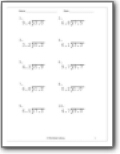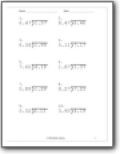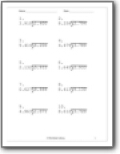# Decimal Division Worksheets

#### How to Divide Decimals

Now that you have grasped the basic concept of decimal, then dividing it is the next important step. Always remember that the trick is to get rid of the decimal point from the dividend. Now, how is that possible? You have to multiply the number by ten until you get a whole number. Let us take an example of 15/0.2. Let us multiply 0.2 by 10, which shifts the point out of the way. 0.2 ×10 = 2. But you must also multiply 10 with the numerator. 15 ×10 = 150.Now, let's try to solve the question. 150/2 = 75. This approach is also best when you have decimals both in numerators and denominators.###### Tenths

Students divide decimal values gauged out to the tenths.###### Hundredths Place

Find the end quotient value between two decimal values that contain a hundredths place.###### Thousandths

We divide values that contain decimal thousandths places.